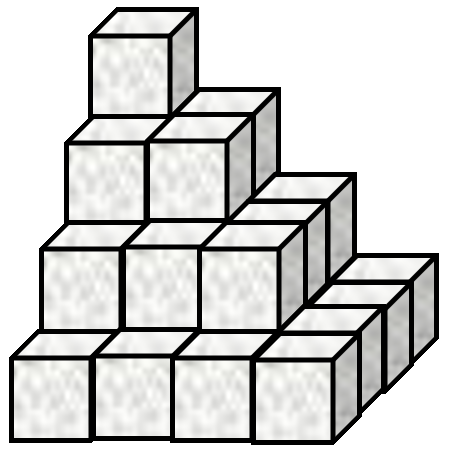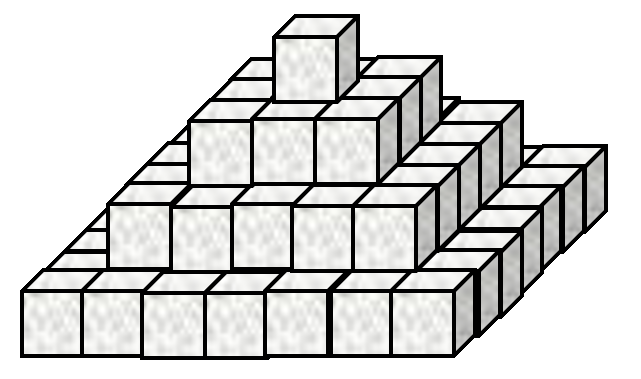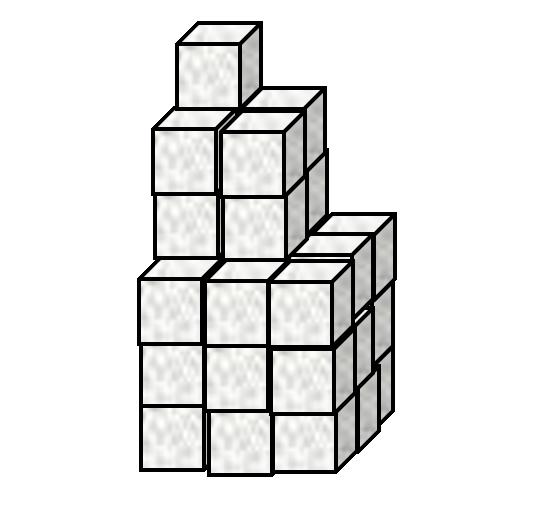# First Steps

It is easy to add lists of numbers together

      1 2 3 + 4 5 6

5 7 9


Negative numbers are written with a high minus ¯ to differentiate between negation ¯3 = -3 and literal negative numbers

      1 2 3 - 1 0 ¯1

0 2 4


## Singleton extension

Dyadic functions (functions with two input arguments, one on the left and one on the right) can map between a single value and an array of values.

      3 × 1 10 100

3 30 300


      3 = 1 2 3 4 5

0 0 1 0 0


## The reduction operator

Adding a list of numbers could become very tedious...

      1+2+3+4+5+6+7+8+9+10+11+12+13+14+15

120


The reduce operator F/ inserts the function F to its left between parts of the right argument array.

      +/1 2 3 4 5 6 7 8 9 10 11 12 13 14 15

120


## The index generator

The index generator ⍳⍵ generates integers up to the integer right argument ⍵

      ⍳10

1 2 3 4 5 6 7 8 9 10


So we can do an arithmetic sum as follows

Traditional Mathematical Notation (TMN) APL
$$\sum_{n=1}^N n$$ +/⍳N

## Order of execution

Infix (dyadic) functions have a short left scope and long right scope.

      10×⍳2+5

10 20 30 40 50 60 70


The expresssion above is "ten times the indices from 1 to two plus five, or in short: "ten times iota two plus five". We can make it clearer using (superfluous) parentheses ().

      10×(⍳(2+5))

10 20 30 40 50 60 70


Of course, we can change the order of execution using different parentheses.

      (10×⍳2)+5

15 25


## Problem Set 1

1. A Mathematical Notation

Use APL to evaluate the following

1. $$\prod_{n=1}^{12} n$$ (multiply together the first twelve integers)

2. $$\sum_{n=1}^{17}n^2$$ (add together the first seventeen squared integers)

3. $$\sum_{n=1}^{100}2n$$ (add together the first one hundred positive even integers)

4. $$\sum_{n=1}^{100}2n-1$$ (add together the first one hundred odd integers)

5. In traditional mathematical notation (TMN), the following equation equals 0, why does the following return 70?

      84 - 12 - 1 - 13 - 28 - 9 - 6 - 15
70

Answers

1. $$\prod_{n=1}^{12} n$$
      ×/⍳12
479001600
2. $$\sum_{n=1}^{17}n^2$$
      +/(⍳17)*2
1785
3. $$\sum_{n=1}^{100}2n$$
      +/2×⍳100
10100
4. $$\sum_{n=1}^{100}2n-1$$
      +/¯1+2×⍳100
10000
5. Expressions are grouped with long right scope and short left scope, rather than using BODMAS. With parentheses, the APL and TMN can be made equivalent:
      84 - (12 - (1 - (13 - (28 - (9 - (6 - 15))))))
70
(((((((84 - 12) - 1) - 13) - 28) - 9) - 6) - 15)
0

2. Pyramid Schemes

1. Sugar cubes are stacked in an arrangement as shown by Figure 1.This stack has 4 layers and a total of 30 cubes. How many cubes are there in a similar stack with 467 layers?

2. Now consider the stack in Figure 2.The arrangement in Figure 2 has 4 layers and 84 cubes. How many cubes are there in a similar stack with 812 layers?

3. Now look at Figure 3.The stack in Figure 3 has 3 "layers" and 36 cubes in total. How many cubes are there in a similar stack with 68 "layers"?

Answers

1.       +/(⍳467)*2
34058310

2.       +/(¯1+2×⍳812)*2
713849500

3.       +/(⍳68)*3
5503716

3. What's in a Vector?
⎕AVU is a list (vector) of numbers (don't worry about what it represents). Find the following properties of ⎕AVU:

1. Find the sum of all the values in ⎕AVU.

2. Find the product of all the values in ⎕AVU.

3. What is the length of ⎕AVU?

4. Find the mean average of ⎕AVU.

Answers

1.       +/⎕AVU
646112

2.       ×/⎕AVU
0
3. There are many ways to compute the number of numbers in a numeric vector. Here are a couple:
      +/⎕AVU=⎕AVU
256
+/⎕AVU*0
256
+/1+⎕AVU×0
256
4. The mean average is the sum divided by the length:
      (+/⎕AVU)÷+/⎕AVU=⎕AVU
2523.875